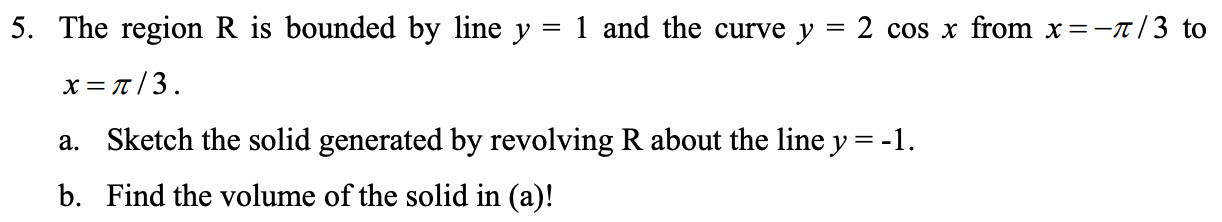### Create an Account

Already have account?

### Forgot Your Password ?

Home / Questions / 5. The region R is bounded by line y = 1 and the curve y = 2 cos x from x=-1/3 to x=a/3. a...

# 5. The region R is bounded by line y = 1 and the curve y = 2 cos x from x=-1/3 to x=a/3. a. Sketch the solid generated by revolving R about the line y=-1. b. Find the volume of the solid in (a)!

5. The region R is bounded by line y = 1 and the curve y = 2 cos x from x=-1/3 to x=a/3. a. Sketch the solid generated by revolving R about the line y=-1. b. Find the volume of the solid in (a)!Apr 14 2021 View more View Less

#### Answer (Solved)Subscribe To Get Solution